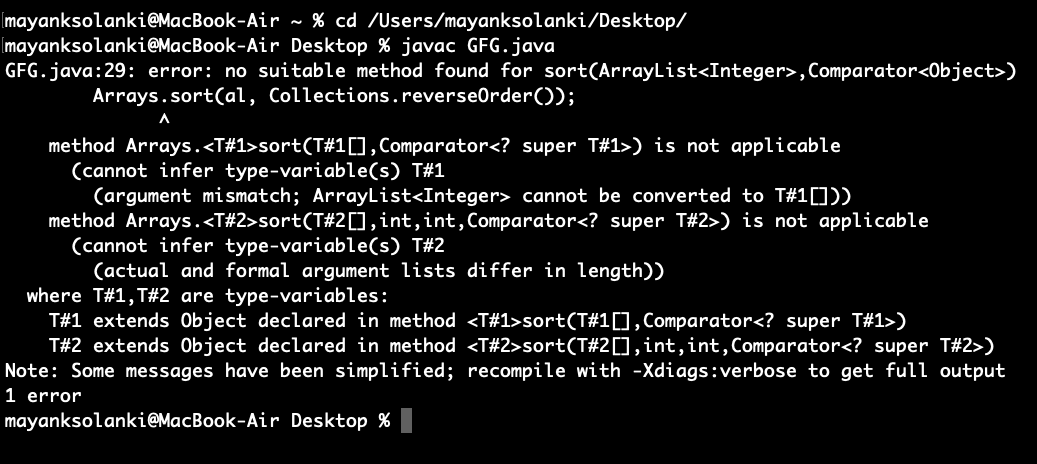Open in App
Not now

# Collections.reverseOrder() in Java with Examples

• Difficulty Level : Easy
• Last Updated : 10 Jan, 2023

The reverseOrder() method of Collections class that in itself is present inside java.util package returns a comparator and using this comparator we can order the Collection in reverse order. Natural ordering is the ordering imposed by the objects’ own compareTo method.

Syntax:

`public static  Comparator reverseOrder()`

Parameter: A comparator whose ordering is to be reversed by the returned comparator(it can also be null)

Return Type: A comparator that imposes the reverse of the natural ordering on a collection of objects that implement the Comparable interface

Now in order to dig deeper to understand to grassroots, we will be covering different use-cases s listed below as follows:

1. To sort a list in descending order
2. To Sort an Array in Descending Order
3. To sort students in descending order of roll numbers when there is a user-defined comparator to do reverse.

Case 1: To sort a list in descending order

Example

## Java

 `// Java Program to Demonstrate Working of reverseOrder()``// method of Collections class``// To sort a list in descending order`` ` `// Importing required utility classes``import` `java.util.*;`` ` `// Main class``// Collectionsorting``public` `class` `GFG {`` ` `    ``// Main driver method``    ``public` `static` `void` `main(String[] args)``    ``{``        ``// Creating a list of integers for which we``        ``// create an empty ArrayList by``        ``// declaring object of ArrayList class``        ``ArrayList al = ``new` `ArrayList();`` ` `        ``// Custom input integer elements``        ``al.add(``30``);``        ``al.add(``20``);``        ``al.add(``10``);``        ``al.add(``40``);``        ``al.add(``50``);`` ` `        ``// Using sort() method of Collections class to``        ``// sort the elements and passing list and using``        ``// reverseOrder() method to sort in descending order``        ``Collections.sort(al, Collections.reverseOrder());`` ` `        ``// Lastly printing the descending sorted list on``        ``// console``        ``System.out.println(``            ``"List after the use of Collection.reverseOrder()"``            ``+ ``" and Collections.sort() :\n"` `+ al);``    ``}``}`

Output

```List after the use of Collection.reverseOrder() and Collections.sort() :
[50, 40, 30, 20, 10]```

Note: Geeks now you must be thinking that can we use Arrays.sort()?

Arrays.sort() cannot be used directly to sort primitive arrays in descending order. If we try to call the Arrays.sort() method by passing reverse Comparator defined by Collections.reverseOrder(), it will throw the error as shown below as follows:Tip: But this will work fine with ‘Array of Objects’ such as the Integer array but will not work with a primitive array such as the int array.

Case 2: To Sort an Array in Descending Order

Example

## Java

 `// Java Program to Demonstrate Working of reverseOrder()``// method of Collections class``// To Sort an Array in Descending Order`` ` `// Importing required utility classes``import` `java.util.*;`` ` `// Main class``// CollectionSorting``public` `class` `GFG {`` ` `    ``// Main driver method``    ``public` `static` `void` `main(String[] args)``    ``{`` ` `        ``// Creating an array to be sorted in descending``        ``// order``        ``Integer[] arr = { ``30``, ``20``, ``40``, ``10` `};`` ` `        ``// Collections.sort method is sorting the``        ``// elements of arr[] in descending order``        ``// later on Arrays.sort() is applied to sort array``        ``Arrays.sort(arr, Collections.reverseOrder());`` ` `        ``// Printing the sorted array on console``        ``System.out.println(``            ``"Array after the use of Collection.reverseOrder()"``            ``+ ``" and Arrays.sort() :\n"``            ``+ Arrays.toString(arr));``    ``}``}`

Output

```Array after the use of Collection.reverseOrder() and Arrays.sort() :
[40, 30, 20, 10]```

Case 3: To sort students in descending order of roll numbers when there is a user-defined comparator to do reverse.

`public static Comparator reverseOrder(Comparator c) `

It returns a Comparator that imposes reverse order of a passed Comparator object. We can use this method to sort a list in reverse order of user-defined Comparator. For example, in the below program, we have created a reverse of the user-defined comparator to sort students in descending order of roll numbers.

Example:

## Java

 `// Java Program to Demonstrate Working of``// reverseOrder(Comparator c)``// To sort students in descending order of roll numbers``// when there is a user defined comparator to do reverse`` ` `// Importing required classes``import` `java.io.*;``import` `java.lang.*;``import` `java.util.*;`` ` `// Class 1``// Helper student class``// to represent a student``class` `Student {``    ``int` `rollno;``    ``String name, address;`` ` `    ``// Constructor``    ``public` `Student(``int` `rollno, String name, String address)``    ``{`` ` `        ``// This keyword refers to current instance itself``        ``this``.rollno = rollno;``        ``this``.name = name;``        ``this``.address = address;``    ``}`` ` `    ``// Method of Student class``    ``// To print student details inside main() method``    ``public` `String toString()``    ``{`` ` `        ``return` `this``.rollno + ``" "` `+ ``this``.name + ``" "``            ``+ ``this``.address;``    ``}``}`` ` `// Class 2``// Helper class implementing interface``class` `Sortbyroll ``implements` `Comparator {`` ` `    ``// Method``    ``// Used for sorting in ascending order of``    ``// roll number``    ``public` `int` `compare(Student a, Student b)``    ``{``        ``return` `a.rollno - b.rollno;``    ``}``}`` ` `// Class 3``// Main class``class` `GFG {`` ` `    ``// Main driver method``    ``public` `static` `void` `main(String[] args)``    ``{`` ` `        ``// Creating an empty ArrayList``        ``ArrayList ar = ``new` `ArrayList();`` ` `        ``// Adding custom attributes defined in Student class``        ``// using add() method``        ``ar.add(``new` `Student(``111``, ``"bbbb"``, ``"london"``));``        ``ar.add(``new` `Student(``131``, ``"aaaa"``, ``"nyc"``));``        ``ar.add(``new` `Student(``121``, ``"cccc"``, ``"jaipur"``));`` ` `        ``// Display message for better readability``        ``System.out.println(``"Unsorted"``);`` ` `        ``// Printing list of students``        ``for` `(``int` `i = ``0``; i < ar.size(); i++)``            ``System.out.println(ar.get(i));`` ` `        ``// Sorting a list of students in descending order of``        ``// roll numbers using a Comparator``        ``// that is reverse of Sortbyroll()``        ``Comparator c``            ``= Collections.reverseOrder(``new` `Sortbyroll());``        ``Collections.sort(ar, c);`` ` `        ``// Display message for better readability``        ``System.out.println(``"\nSorted by rollno"``);`` ` `        ``// Printing sorted students in descending order``        ``for` `(``int` `i = ``0``; i < ar.size(); i++)``            ``System.out.println(ar.get(i));``    ``}``}`

Output:

```Unsorted
111 bbbb london
131 aaaa nyc
121 cccc jaipur

Sorted by rollno
131 aaaa nyc
121 cccc jaipur
111 bbbb london```

The key thing here to remember is above program uses unchecked and unsafe operations.

This article is contributed by Mohit Gupta. If you like GeeksforGeeks and would like to contribute, you can also write an article using write.geeksforgeeks.org or mail your article to review-team@geeksforgeeks.org. See your article appearing on the GeeksforGeeks main page and help other Geeks.

My Personal Notes arrow_drop_up